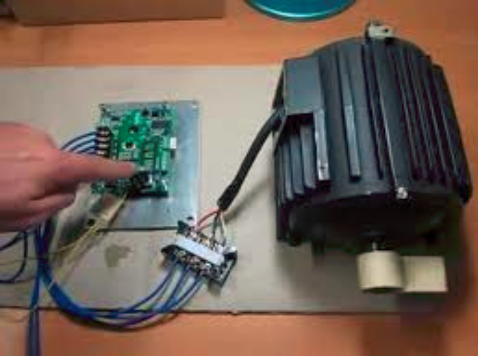HOMEBLOG & NEWSIndustry News# How to Determine an AC Induction Motor Speed

By 本站 2017/11/29 15:46:30

Matching motor how to determine the motor speed? The motor will explain your choice how to determine the motor speed motor:

Rated speed of the motor selection, according to the requirements of the production machinery and the driving device to consider the ratio of the transmission device.

Revolutions per minute, the motor usually has 3000 1500, 1000, 750 and 600 of the rated speed of asynchronous motor due to slip, to the low speed of 2% ~ 5%.

From the perspective of motor manufacturing, motor with same power if the rated speed is high, the electromagnetic torque size is small, the cost is low and light weight, and high speed motor power factor and efficiency are higher than low speed motor. If the motor can choose higher speed, then the economy is better, but if the resulting motor and the driven machine of the speed difference is too large, the drive series required installed speed reducer is more, it will increase the cost of equipment and energy loss in transmission. Therefore, through the analysis and comparison of selected.

We usually use most of the motor is the use of 4 pole 1500r/min motor, because the rated speed of the motor to adapt to a wide range, and its power factor and work efficiency is also higher.In most cases, you can look inside the motor and count the number of poles in the winding; they are distinct bundles of wire evenly spaced around the stator core.

The number of poles, combined with the ac line frequency (Hertz, Hz), are all that determine the no-load revolutions per minute (rpm) of the motor. So, all four-pole motors will run at the same speed under no-load conditions, all six-pole motors will run at the same speed, and so on.

The mathematical formula to remember in helping make this calculation is the number of cycles (Hz) times 60 (for seconds in a minute) times two (for the positive and negative pulses in the cycle) divided by the number of poles.
Therefore, for a 60-Hz system, the formula would be:
60 x 60 x 2 = 7,200 no-load rpm Ã· number of poles.
For a 50-Hz system, the formula would be:
50 x 60 x 2 = 6,000 no-load rpm Ã· number of poles.

Using this formula, you can see that a four-pole motor operating on the bench under no-load conditions runs at 1,800 rpm (7,200 Ã· 4 poles). Note that when an ac motor is loaded, the spinning magnetic field in the stator does not change speed. Instead, the rotor or moving part of the motor is restrained by the load from “catching up” to the field speed.

The difference between the field speed of 1,800 rpm in this example and the rotor speed of approximately 1,725 rpm is called the “slip.” Slip varies with the load over a narrow operating range for each motor design.

The above content according to arrangement of the practical problems encountered in the work and, for reference, if there is a problem, please communicate with customer service: sales@doulipower.com.

Share：